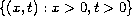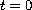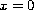Electron. J. Diff. Eqns., Vol. 2008(2008), No. 157, pp. 1-8.

### Explicit solutions for a system of first-order partial differential equations Kayyunnapara Thomas Joseph

Abstract:
In this paper we construct explicit weak solutions of a system of two partial differential equations in the quarter planewith initial conditions atand a weak form of Dirichlet boundary conditions at. This system was first studied by LeFloch , where he constructed explicit formula for the weak solution of pure initial value problem.

Submitted November 1, 2008. Published November 20, 2008.
Math Subject Classifications: 35A20, 35L50, 35R05.
Key Words: First order equations; boundary conditions; exact solutions

Show me the PDF file (208 KB), TEX file, and other files for this article.Kayyunnapara Thomas Joseph School of Mathematics Tata Institute of Fundamental Research Homi Bhabha Road Mumbai 400005, India email: ktj@math.tifr.res.in